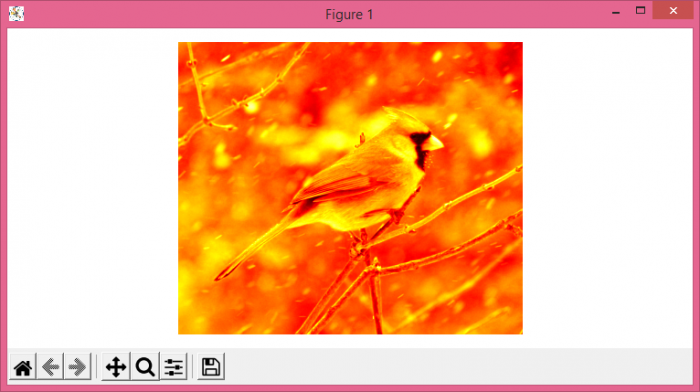# Set a colormap of an image in Matplotlib

To set a colormap of an image, we can take the following steps −

• Set the figure size and adjust the padding between and around the subplots.
• Read an image from a file into an array.
• Pick one channel of your data.
• Display the data as an image, i.e., on a 2D regular raster with "hot" colormap
• Turn off the axes.
• To display the figure, use show() method.

## Example

from matplotlib import pyplot as plt, image as mimg

plt.rcParams["figure.figsize"] = [7.50, 3.50]
plt.rcParams["figure.autolayout"] = True

lum_img = img[:, :, 0]

plt.imshow(lum_img, cmap="hot")

plt.axis('off')

plt.show()

## Output